# KSEEB Solutions for Class 9 Maths Chapter 6 Constructions Ex 6.2

In this chapter, we provide KSEEB SSLC Class 9 Maths Chapter 6 Constructions Ex 6.2 for English medium students, Which will very helpful for every student in their exams. Students can download the latest KSEEB SSLC Class 9 Maths Chapter 6 Constructions Ex 6.2 pdf, free KSEEB SSLC Class 9 Maths Chapter 6 Constructions Ex 6.2 pdf download. Now you will get step by step solution to each question.

## Karnataka Board Class 9 Maths Chapter 6 Constructions Ex 6.2

Question 1.
Construct a triangle ABC in which BC = 7 cm, ∠B = 75° and AB + AC = 13 cm,
Solution:
Construction of a triangle ABC in which BC = 7 cm, ∠B = 75° and AB + AC = 13 cm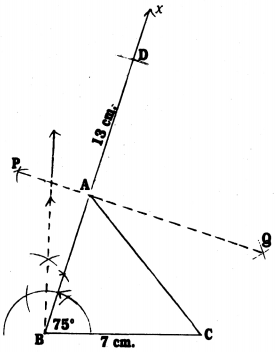Steps of Construction :

1. Draw BC = 7 cm line segment.
2. Construct ∠B = 75° and produce it upto Bx.
3. With B as centre draw an arc BD = 13 cm such that it intersects Bx at D.
4. Construct PQ which is the perpendicular bisector of BD.
5. PQ intersects BD at A.
6. Join AC.
7. Now ∆ABC, with measurement AB + AC = 13 cm, and ∠B = 75°, BC = 7 cm is constructed.

Question 2.
Construct a triangle ABC in which BC = 8 cm, ∠B = 45° and AB – AC = 3.5 cm.
Solution:
Constructing a triangle ABC in which BC = 8 cm, ∠B = 45° and AB – AC = 3.5 cm.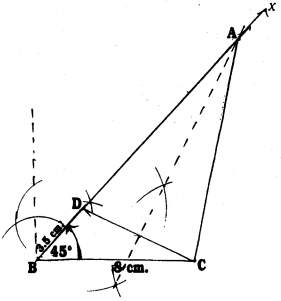Steps of Construction :

1. Draw a line segment BC = 8 cm.
2. Draw ∠B = 45° with the help of a protractor. Bx is joined.
3. Draw an arc BD = 3.5 cm.
4. When perpendicular bisector DC is drawn, that intersects Bx at A. AC is joined.
5. Now, DABC with BC = 8 cm, ∠B = 45° and AB – AC = 3.5 cm. is constructed.

Question 3.
Construct a triangle PQR in which QR = 6 cm, ∠Q = 60° and PR – PQ = 2 cm.
Solution:
Constructing a triangle PQR in which QR = 6 cm, ∠Q = 60° and PR – PQ = 2 cm.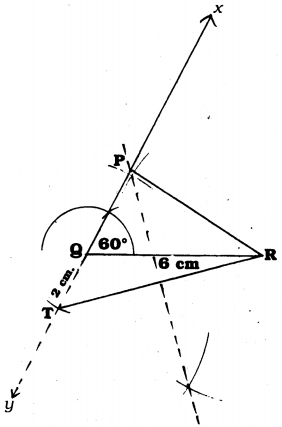Steps of Construction :

1. Draw a line segment QR = 6 cm.
2. With the help of a protractor draw a line segment Qx and ∠Q = 60°.
3. Produce line segment xQ upto y. mark T such that QT = 2 cm. Join TR.
4. If the perpendicular bisector of TR is drawn, that meets line segment Qx at P. PR is joined.
Now, ∆PQR is constructed.

Question 4.
Construct a triangle XYZ in which ∠Y = 30°, ∠Z = 90° and XY + YZ + ZX = 11 cm.
Solution:
Constructing a triangle XYZ in which ∠Y = 30°, ∠Z = 90° and XY + YZ + ZX = 11 cm.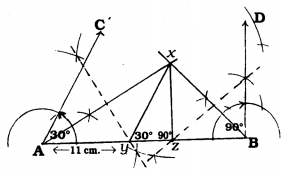Steps of Construction :

1. Draw AB = 11 cm line segment with measurement XY + YZ + ZX.
2. With the help of protractor construct ∠A = 30°, ∠B = 90°.
3. Construct angular bisectors of ∠CAB and ∠DBA which meet at x. Ax, Bx are joined.
4. If perpendicular bisector AX is drawn, it intersects AB at Y and AB at Z.
5. By joining XY and XZ, ∆XYZ is constructed.

Question 5.
Construct a right triangle whose base is 12 cm and the sum of its hypotenuse and the other side is 18 cm.
Solution:
Constructing a right-angled triangle ABC with base, AB =12 cm and the sum of hypotenuse BC and another side AC, AC + BC = 18 cm.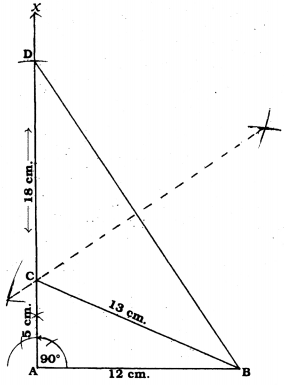Steps of Construction :

1. Draw a line segment AB = 12 cm.
2. With the help of a protractor construct ∠A = 90° and produce upto AX.
3. Mark ‘D’ on AX such that AD = 18 cm.
4. Join BD.
5. Let the perpendicular bisector BD intersects AD at C. Join BC.
6. Now required triangle ABC is constructed with Base AB = 12cm, Side AC = – 5 cm, Hypotenuse BC = 13 cm and ∠A = 90°.

All Chapter KSEEB Solutions For Class 9 Maths

—————————————————————————–

All Subject KSEEB Solutions For Class 9

*************************************************

I think you got complete solutions for this chapter. If You have any queries regarding this chapter, please comment on the below section our subject teacher will answer you. We tried our best to give complete solutions so you got good marks in your exam.

If these solutions have helped you, you can also share kseebsolutionsfor.com to your friends.

Best of Luck!!